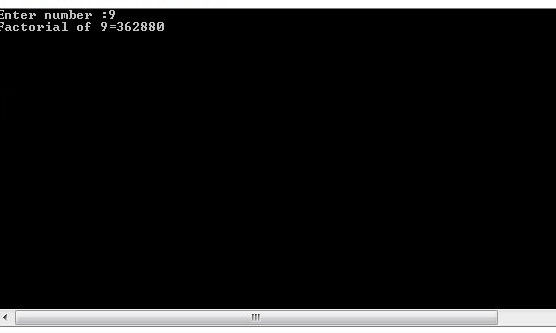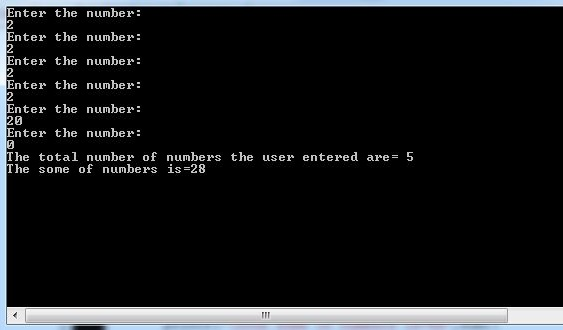# Learning Do while LOOP

In this part you will learn: 1. Do while LOOP 2. C syntax 3. Showing output In this tutorial I will teach you how to use do while loops in C. We have already covered for loops and now we know what are loops. In this tutorial I will teach you how to use a Do while loop in C. We have previously covered how to use a and now we know how loops work. What is a DO WHILE LOOP? A Do while loop is somewhat similar to a While loop. It has there necessary statements initialization, condition and increment. The initialization is done outside the body of the loop, the condition comes with the while part in round brackets and increment is done inside the body of the loop. In a do while loop the body of the loop is executed first and then afterwards the condition of the loop is tested. If it is true, the code executes the body of the loop again. Calculating Factorial Basic Step: Open Dev C++ then File > new > source file and start writing the code below.
`#include<stdio.h>#include<conio.h>`
These are the header files that we need for coding. The main header is stdio.h which provides us with most of the functions for coding in C and the second header files is just for using a function which can pause the screen until the user hits any key.
`int main(){int num,fact=1;`
For finding the factorial of a number we take input of a number and Inside the loop, we multiply the counter times the accumulated number up to that point. In this case the accumulator is ‘fact’.
`	printf("Enter number :");	scanf("%d",&num);	int i=num;`
Now taking input from the user.
`do{fact=fact*num;        	num--;		}while(num>0); }`
Now we write the do while loop. Note that we are multiplying the number we entered with ‘fact’ which is the accumulator and initialized to 1. Then we made a decrement in the number and after that we checked it with the condition. Our number should always be greater the 0 in any case. We copied the value of number into a variable ‘i’ just to print the original number.
`	printf("Factorial of %d=%d\n",i,fact);	getch();	return 0;}`
Execute > compile then Execute > run OutputSUM OF ALL THE NUMBERS UNTIL USER ENTER ZERO File > new source file
`#include<stdio.h> int main(){	Int sum=0,num,totalnumbers=0;  `
For calculating the sum of all the numbers the user entered we need a variable to store the numbers user enter. Then we need a variable ‘sum’ to accumulate the sum of numbers. And we need a variable to keep the record of total numbers the user entered.
`do{       printf("Enter the number:\n");       scanf("%d",&num);        totalnumbers++;        sum=sum+num;       }while(num!=0); `
Now we write the body of the loop which takes a number from user and adds it to the accumulator. Then the condition checks if the user has entered 0 it should stop taking any more numbers from the user and break out of the loop. Otherwise it should keep going on taking numbers from the user and adding them.
`   totalnumbers--;   printf("The total number of numbers the user entered are=     %d",totalnumbers);   printf("\nThe some of numbers is=%d",sum); getch();`
Lastly we printed the total number of numbers user entered except the 0 at the end. We should not count the 0 because its our exit condition. And we printed the sum. Execute > compile then Execute > run Output: